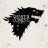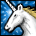## How to change the i.MX28 LCD Dotclk Frequency from 24 MHz to 30 MHz? When enter standby mode how to change the VSYNC and HSYNC from High to Low for decrease the LCD leak current?

cancel
Showing results for
Did you mean:
SOLVED

## How to change the i.MX28 LCD Dotclk Frequency from 24 MHz to 30 MHz? When enter standby mode how to change the VSYNC and HSYNC from High to Low for decrease the LCD leak current?

1,045 ViewsContributor I

How to change the i.MX28 LCD Dotclk Frequency from 24 MHz to 30 MHz? When enter standby mode how to change the VSYNC and HSYNC from High to Low for decrease the LCD leak current?

Now in my project, when exit the standby mode the LCD will twinking at the first seconds, after the first seconds the LCD will return to normal, I want to increase the LCD Dotclk frequency from 24 MHz to 30 MHz but the frequency still is 24 MHz. Can you inform me how to change the LCD Dotclk frequency? And I check when exit the standby mode the LCD Dotclk frequency will change from a very fast frequency to 24 MHz, so I think maybe the LCD twinking problem cause by the very fast frequency. Have you any hints?

In addition, for decrease the standby mode curret, we also want to change the VSYNC and HSYNC from High to Low when enter the standby mode, I try to change the register for the VSYNC_POL and HSYNC_POL, but still have not successful. Have you give me help for these issues! Thanks all of you in advance!

Labels (1)
• ### i.MX2x

Tags (2)
1 Solution
179 ViewsContributor II

The LCD dotclk frequency is hardcoded in the program. Furthermore, for some unknown reason, the program chooses to use BYPASS mode for LCD clock. In other words, the maximum frequency is only 24MHz.

Here is my implementation of lcdif_set_rate in arch/arm/mach-mxs/clock.c. The clock rate will be chosen based on setting of fb_entry.cycle_time_ns (in drivers/video/mxs/lcd_43wvf1g.c).

//----------------------

static int lcdif_set_rate(struct clk *clk, unsigned long rate)
{
u32 cycle_time = 1000000 / rate;  // in ns (rate is in KHz)
int n, div, err;
int reg_val;

int min_err=9999, min_err_n=0, min_err_div=0;

for (n=18; n < 36; n++) {    // possible value (18..35) for fractional clock control divider
// Freq after fractional divider ffreq = 480MHz * 18 / n
//
// Further divide required = cycle_time_in_s / ( 1/ ffreq) = cycle_time_in_ns / (1000 n / (480 * 18)) = cycle_time * 216 / 25n
// Formula used rounds the divisor to nearest interger.
div = ((cycle_time * 2 * 216) / (25 * n) + 1) >> 1;
if (div >= 256) continue;    // suggested value is from 1 to 255
err = div * 25 * n - cycle_time * 216;
if (err < 0) err = -err;

if (err < min_err) {
min_err = err;
min_err_n = n;
min_err_div = div;
}
}
if (min_err >= 9999) return -EINVAL;

/* Program ref_pix phase fractional divider */
reg_val &= ~BM_CLKCTRL_FRAC1_PIXFRAC;
reg_val |= BF_CLKCTRL_FRAC1_PIXFRAC(min_err_n);

/* Ungate PFD */
__raw_writel(BM_CLKCTRL_FRAC1_CLKGATEPIX,

reg_val &= ~(BM_CLKCTRL_DIS_LCDIF_DIV | BM_CLKCTRL_DIS_LCDIF_CLKGATE);
reg_val |= (min_err_div << BP_CLKCTRL_DIS_LCDIF_DIV) & BM_CLKCTRL_DIS_LCDIF_DIV;
__raw_writel(reg_val, clk->scale_reg);
if (clk->busy_reg) {
int i;
for (i = 10000; i; i--)
if (!clk_is_busy(clk))
break;
if (!i)
return -ETIMEDOUT;
}

/* Switch to ref_pix source */
reg_val &= ~BM_CLKCTRL_CLKSEQ_BYPASS_DIS_LCDIF;

return 0;
}
// -----------------------------

3 Replies
180 ViewsContributor II

The LCD dotclk frequency is hardcoded in the program. Furthermore, for some unknown reason, the program chooses to use BYPASS mode for LCD clock. In other words, the maximum frequency is only 24MHz.

Here is my implementation of lcdif_set_rate in arch/arm/mach-mxs/clock.c. The clock rate will be chosen based on setting of fb_entry.cycle_time_ns (in drivers/video/mxs/lcd_43wvf1g.c).

//----------------------

static int lcdif_set_rate(struct clk *clk, unsigned long rate)
{
u32 cycle_time = 1000000 / rate;  // in ns (rate is in KHz)
int n, div, err;
int reg_val;

int min_err=9999, min_err_n=0, min_err_div=0;

for (n=18; n < 36; n++) {    // possible value (18..35) for fractional clock control divider
// Freq after fractional divider ffreq = 480MHz * 18 / n
//
// Further divide required = cycle_time_in_s / ( 1/ ffreq) = cycle_time_in_ns / (1000 n / (480 * 18)) = cycle_time * 216 / 25n
// Formula used rounds the divisor to nearest interger.
div = ((cycle_time * 2 * 216) / (25 * n) + 1) >> 1;
if (div >= 256) continue;    // suggested value is from 1 to 255
err = div * 25 * n - cycle_time * 216;
if (err < 0) err = -err;

if (err < min_err) {
min_err = err;
min_err_n = n;
min_err_div = div;
}
}
if (min_err >= 9999) return -EINVAL;

/* Program ref_pix phase fractional divider */
reg_val &= ~BM_CLKCTRL_FRAC1_PIXFRAC;
reg_val |= BF_CLKCTRL_FRAC1_PIXFRAC(min_err_n);

/* Ungate PFD */
__raw_writel(BM_CLKCTRL_FRAC1_CLKGATEPIX,

reg_val &= ~(BM_CLKCTRL_DIS_LCDIF_DIV | BM_CLKCTRL_DIS_LCDIF_CLKGATE);
reg_val |= (min_err_div << BP_CLKCTRL_DIS_LCDIF_DIV) & BM_CLKCTRL_DIS_LCDIF_DIV;
__raw_writel(reg_val, clk->scale_reg);
if (clk->busy_reg) {
int i;
for (i = 10000; i; i--)
if (!clk_is_busy(clk))
break;
if (!i)
return -ETIMEDOUT;
}

/* Switch to ref_pix source */
reg_val &= ~BM_CLKCTRL_CLKSEQ_BYPASS_DIS_LCDIF;

return 0;
}
// -----------------------------

179 ViewsContributor II

Great ! It works on my TFT 7'' with 30 MHz typical dotclock

179 ViewsContributor V

Conrad, thanks for sharing. This has saved me a lot of time .......

Gonzalo.